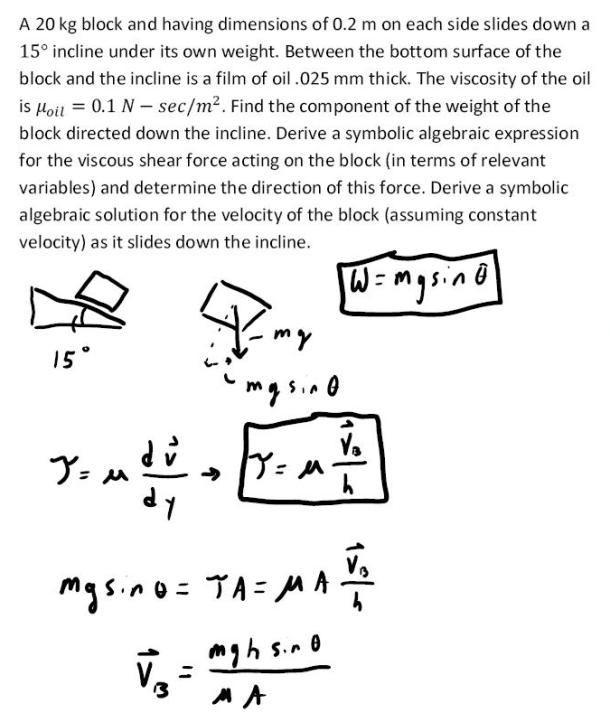fluid mechanics math problems engineering equations fluid mechanics basic mechanics school homework engineering math fluids formulas fluid mechanics problems fluid mechanics problem solutions to fluids problems full solution fluid mechanics engineering fluids problem solution fluid mechanics math problems engineering equations fluid mechanics basic mechanics school homework engineering math fluid mechanics formulas fluid problems fluid mechanics problem solutions to fluid mechanics problems full solution fluid mechanics engineering fluid mechanics problem solution
fluid mechanics math problems engineering equations fluid mechanics basic mechanics school homework engineering math fluids formulas fluid mechanics problems fluid mechanics problem solutions to fluids problems full solution fluid mechanics engineering fluids problem solution fluid mechanics math problems engineering equations fluid mechanics basic mechanics school homework engineering math fluid mechanics formulas fluid problems fluid mechanics problem solutions to fluid mechanics problems full solution fluid mechanics engineering fluid mechanics problem solution
Highalphabet Home Page fluid mechanics solutions fluids math solved Fluid Mechanics Page 1
A 20 kg block and having dimensions of .2 m on each side slides down a 15 degree incline under its own weight. Between the bottom surface of the block and the incline is a film of oil .025 mm thick. The viscosity of the oil is .1 N-sec/m^2. Find the component of the weight of the block directed down the incline. Derive a symbolic algebraic expression for the viscous shear foce acting on the block (in terms of relevant variables) and determine the direction of this force. Derive a symbolic algebraic soluution for the velocity of the block (assuming constant velocity) as it slides down the inlcine.A 20 kg block and having dimensions of .2 m on each side slides down a 15 degree incline under its own weight. Between the bottom surface of the block and the incline is a film of oil .025 mm thick. The viscosity of the oil is .1 N-sec/m^2. Find the component of the weight of the block directed down the incline. Derive a symbolic algebraic expression for the viscous shear foce acting on the block (in terms of relevant variables) and determine the direction of this force. Derive a symbolic algebraic soluution for the velocity of the block (assuming constant velocity) as it slides down the inlcine.